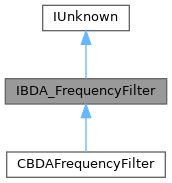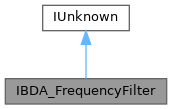ReactOS  0.4.15-dev-3717-g292e6a4
IBDA_FrequencyFilter Interface Reference

import"bdaiface.idl";

Inheritance diagram for IBDA_FrequencyFilter:[legend]
Collaboration diagram for IBDA_FrequencyFilter:[legend]

Public Member Functions

HRESULT put_Autotune ([in] ULONG ulTransponder)

HRESULT get_Autotune ([in, out] ULONG *pulTransponder)

HRESULT put_Frequency ([in] ULONG ulFrequency)

HRESULT get_Frequency ([in, out] ULONG *pulFrequency)

HRESULT put_Polarity ([in] Polarisation Polarity)

HRESULT get_Polarity ([in, out] Polarisation *pPolarity)

HRESULT put_Range ([in] ULONG ulRange)

HRESULT get_Range ([in, out] ULONG *pulRange)

HRESULT put_Bandwidth ([in] ULONG ulBandwidth)

HRESULT get_Bandwidth ([in, out] ULONG *pulBandwidth)

HRESULT put_FrequencyMultiplier ([in] ULONG ulMultiplier)

HRESULT get_FrequencyMultiplier ([in, out] ULONG *pulMultiplier)Public Member Functions inherited from IUnknown
HRESULT QueryInterface ([in] REFIID riid, [out, iid_is(riid)] void **ppvObject)

ULONG Release ()Public Types inherited from IUnknown
typedef IUnknownLPUNKNOWN

Detailed Description

Definition at line 340 of file bdaiface.idl.

◆ get_Autotune()

 HRESULT IBDA_FrequencyFilter::get_Autotune ( [in, out] ULONG * pulTransponder )

Implemented in CBDAFrequencyFilter.

◆ get_Bandwidth()

 HRESULT IBDA_FrequencyFilter::get_Bandwidth ( [in, out] ULONG * pulBandwidth )

Implemented in CBDAFrequencyFilter.

◆ get_Frequency()

 HRESULT IBDA_FrequencyFilter::get_Frequency ( [in, out] ULONG * pulFrequency )

Implemented in CBDAFrequencyFilter.

◆ get_FrequencyMultiplier()

 HRESULT IBDA_FrequencyFilter::get_FrequencyMultiplier ( [in, out] ULONG * pulMultiplier )

Implemented in CBDAFrequencyFilter.

◆ get_Polarity()

 HRESULT IBDA_FrequencyFilter::get_Polarity ( [in, out] Polarisation * pPolarity )

Implemented in CBDAFrequencyFilter.

◆ get_Range()

 HRESULT IBDA_FrequencyFilter::get_Range ( [in, out] ULONG * pulRange )

Implemented in CBDAFrequencyFilter.

◆ put_Autotune()

 HRESULT IBDA_FrequencyFilter::put_Autotune ( [in] ULONG ulTransponder )

Implemented in CBDAFrequencyFilter.

◆ put_Bandwidth()

 HRESULT IBDA_FrequencyFilter::put_Bandwidth ( [in] ULONG ulBandwidth )

Implemented in CBDAFrequencyFilter.

Referenced by CScanningTunner::SetFrequency().

◆ put_Frequency()

 HRESULT IBDA_FrequencyFilter::put_Frequency ( [in] ULONG ulFrequency )

Implemented in CBDAFrequencyFilter.

Referenced by CScanningTunner::SetFrequency().

◆ put_FrequencyMultiplier()

 HRESULT IBDA_FrequencyFilter::put_FrequencyMultiplier ( [in] ULONG ulMultiplier )

Implemented in CBDAFrequencyFilter.

Referenced by CScanningTunner::SetFrequency().

◆ put_Polarity()

 HRESULT IBDA_FrequencyFilter::put_Polarity ( [in] Polarisation Polarity )

Implemented in CBDAFrequencyFilter.

Referenced by CScanningTunner::SetFrequency().

◆ put_Range()

 HRESULT IBDA_FrequencyFilter::put_Range ( [in] ULONG ulRange )

Implemented in CBDAFrequencyFilter.

Referenced by CScanningTunner::SetFrequency().

The documentation for this interface was generated from the following file: